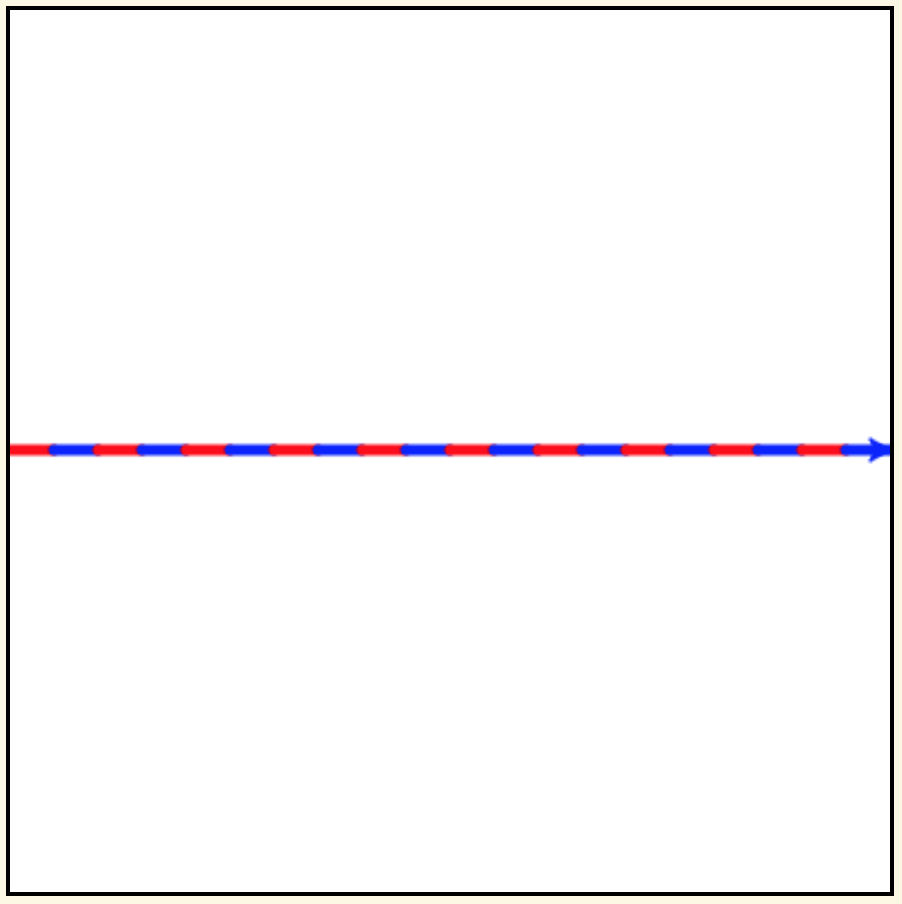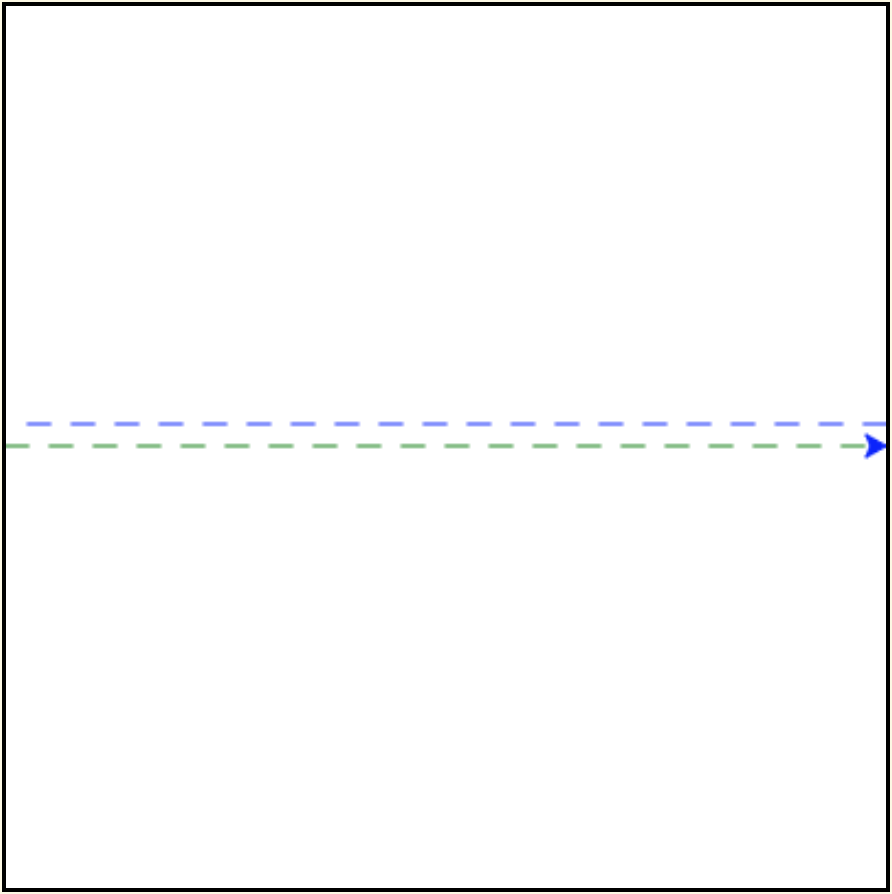# 3.4. Learning to Code¶

## 3.4.1. Variables and assignment¶

A variable is a name that you use in your program to stand for a data object. You should choose meaningful names. For example, you might use `radius` to stand for the length to make the radius of a circle and `area` to stand for its area. You use variables in your program to form expressions. Informally, an expression is just code that an interpreter can evaluate to produce a data object. For example, if `radius` stands for `50`, then evaluating `2 * radius` produces `100`–the diameter of a circle of radius `50`.

In Python, a variable name must start with a letter (`a``z`, `A``Z`) or an underscore (`_`), and can contain only letters, digits (`0``9`) and underscores.

An assignment command has the form `var = expression` where `var` stands for a variable name and `expression` stands for an expression. Executing the assignment makes `var` stand for the data object produced by evaluating `expression`. After the assignment, `var` can be used as a “stand in” for this data object. The data object that a variable stands for is also called the value of the variable.

A variable is like a label for a container (e.g., a file folder) that can hold just one object. In this analogy, an assignment to the variable is like putting something in this container. The term “variable” is used because the value can change—since only one object fits in the container (variable), assigning it a new object (value) replaces any object (value) already in it.

We illustrate this analogy using Python Tutor, a tool for visualizing how an interpreter executes a Python program. It lets you step through your program one line at a time and shows you how the values of variables change.

Press the `Next` button below the code window and see how Python Tutor shows what is going on in computer memory.

Activity: CodeLens 3.4.1.2 (circle_calculations)

You will learn a lot more about variables and assignment on your programming journey. But for today, we’ll let Mike introduce you to one other important notion—the data type of a value—and go on to introducing loops.

One thing in this rap is different for Python: In Python, you declare a variable just by assigning a value to it—you don’t indicate the datatype. Rather, the interpreter figures out the datatype based on how the value is created.

We won’t go into further details now since we can do a lot in Turtle Graphics with just a basic understanding of these notions.

## 3.4.2. Loops¶

Do you ever get tired of repeating the same instructions over and over and over and over and over and … over again? Or, after too many repetitions, do you start making mistakes?

One good thing about computers is that they don’t! Loops are instructions that tell the interpreter to repeat a section of code as many times as needed. Python has two kinds of loop instructions–`for` loops and `while` loops.

The `for` loop:

Recall the example program from last week to draw a square? A skeleton versed in Python will mutter to itself…Why? Because the code we wrote repeats the same two instructions four times in a row. A `for` loop is meant exactly for such situations.

Our skeleton would write this program as shown below. Run this code to see that it draws a square.

The moral: If you can figure out the number of times the interpreter should repeat some code, then use a `for` loop!

The simplest kind of `for` loop has the general form:

`for var in range(int_exp):`
`loop_body_code`

where `var` stands for a variable, `int_exp` stands for an integer expression (an expression that produces an integer when evaluated), and `loop_body_code` stands for code that should be executed `int_exp` number of times.

Some important terminology and rules:

• The words `for` and `in` are called keywords. We’ll learn lots more keywords in the meetings ahead. Never use a keyword for the name of a variable because the interpreter uses keywords to figure out what kind of instruction you want it to execute.

• The code `loop_body_code` is called the body of the loop. It consists of one or more Python commands. Each command in the body must be indented by the same number of spaces. The interpreter tells where the body of a loop starts and ends by the indentation.

Code Along

Replace the last comment with a for-loop that draws an equilateral triangle (with side-lenth 200 pixels).Use the code blocks on the left to construct a program that draws the figure above. Be careful of indentation.Use the code blocks on the left to construct a program that draws the figure above.

Additional important terminology and evaluation rules:

• `range` is the name of a Python standard library function. We’ll learn about functions next week. For now, you just need to know that evaluation of `range(int_exp)` produces a sequence of `int_exp` integers. Specifically, it produces the sequence: `0`, `1`, `2`, … , `int_exp - 1`. (In computer science, it is convenient to start counting at `0` instead of `1`.)

• The variable in the first line of a `for` loop is called the loop variable.

• Each execution of the loop body is called an iteration of the loop.

• Just before each iteration, the interpreter assigns a value from `range(int_exp)` to `var`, starting with `0` and in increasing order.

The last of these rules allows the commands in the loop body to depend on the iteration being executed. This next example illustrates this idea.

Check your understanding of this example by answering the following.

The Sandbox

Use the active code window below as a sandbox in which to play to learn more about coding. (Don’t forget to import `turtle`!)

You have attempted of activities on this page